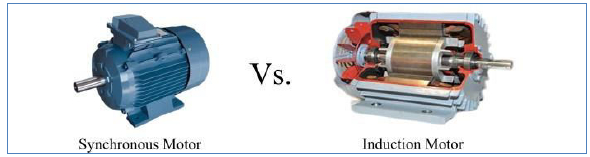# Difference between Synchronous Motor and Induction Motor

A motor in general is an electrical machine that converts electrical energy into mechanical energy. Electric motors can either be DC Motors or AC Motors, depending on the type of power supply that is supplied as its input.

AC motors are further classified into two types−

• Asynchronous or Induction Motor
• Synchronous Motor

A synchronous motor always runs at synchronous speed, while an induction motor runs at a speed less than the synchronous speed.## What is a Synchronous Motor?

A synchronous motor is a type of AC motor in which the rotor rotates at a synchronous speed at all loads, provided that the load on the motor does not exceed the limiting value. If the load on the shaft of a synchronous motor exceeds the limiting value, then the motor comes to rest and the average torque developed by it becomes zero.

A synchronous motor has a stator and a rotor. The stator consists of a 3-phase armature winding and receives power from a balanced 3- phase supply, while the rotor has a set of salient poles excited by direct current to produce alternate N and S poles. In case of a synchronous motor, the stator is wound for the same number of poles as the rotor poles.

## What is an Induction Motor?

An induction motor is a type of AC motor in which the rotor rotates at a speed slightly less than the synchronous speed. Let's see how an induction motor works.

• An induction motor consists of a stator and a rotor. The stator carries a 3-phase winding, while the rotor carries a short-circuited winding.

• When the stator winding is energized from a 3-phase supply, a rotating magnetic field is produced which rotates around the stator.

• This rotating magnetic field passes through the air-gap and cuts the rotor conductors, which are stationary. Therefore, due to relative speed between the rotating magnetic field and the stationary rotor conductors, an EMF is induced in the rotor conductors.

• As the rotor circuit is short-circuited, currents start flowing in the rotor conductors.

• The current carrying rotor conductor are placed in the magnetic field produced by the stator winding. As a result, a torque develops on the rotor conductors and the sum of the torque on all the rotor conductors, rotates the rotor in the same direction as the rotating magnetic field but at a speed slightly less than the synchronous speed.

An induction motor cannot run at synchronous speed. If it runs at synchronous speed, there would be no cutting of the flux by the rotor conductors and there would be no induced EMF, no current and no torque. Therefore, the rotor of the induction motor rotates at a speed slightly less than the synchronous speed. For this reason, an induction motor is also known as an asynchronous motor.

## Difference between Synchronous Motor and Induction Motor

The following table highlights the major points that differentiate a Synchronous Motor from an Induction Motor −

KeySynchronous MotorInduction Motor
Type of MachineA synchronous motor is a doubly excitation machine, i.e., its armature winding is connected to an AC source and its field winding is excited from a DC source.An induction motor is a singly excited machine, that is, its stator winding is energized from an AC source.
SpeedIts speed is independent of the load.Its speed decreases with the increase in load.
StartingIt is not self-starting. It requires external means for starting.Induction motor has self-starting torque.
EfficiencyA synchronous motor is more efficient than induction motor of the same rating.The efficiency of an induction motor is lesser than that of a synchronous motor of same rating.
Power FactorA synchronous motor can operate under a wide range of power factors, both lagging and leading.
The power factor of a synchronous motor can be changed by changing its excitation.
An induction motor operates at only lagging power factor.
The power factor of induction motor cannot be controlled. It becomes very poor (lagging) at high loads.
Relative MotionNo relative motion between the stator rotating magnetic field (RMF) and the rotor is required for the operation of a synchronous motor.For the operation of an induction motor, there must be a relative motion between the stator RMF and the rotor.
Cost-effectivenessFor the same rating, a synchronous motor is expensive than an induction motor.An induction motor is cheaper than a synchronous motor.
ConstructionA synchronous motor has complicated construction.An induction motor have simple construction than a synchronous motor.
Starting TorqueA synchronous motor has high starting torque as compared to an induction motor.An induction motor has less starting torque.
RPMSynchronous motors are economical for speeds below 300 RPM.The induction motors are economical for speeds above 600 RPM.
ExcitationSynchronous motors require DC excitation at the rotor.Induction motors do not require excitation for the rotor.
ApplicationsDriving mechanical loads at constant speed, power factor correction of electrical systems, etc.Induction motors are used for driving mechanical loads only.

## Conclusion

The most important point that you should note here is that the rotors of a Synchronous motor rotates at a synchronous speed, while the rotors in an Asynchronous motor rotates at a speed slightly less than the synchronous speed.